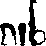# Sinclair Cambridge Programmable - factorial

Factorial n is the product n! = n * (n-1) * (n-2) * ... * 2 * 1

Examples: 1! = 1; 2! = 2; 3! = 6; 6! = 720; 100! ~= 9.333e157.

In a simple language like BASIC you'd write:

10 INPUT N
20 T=N
30 IF N<3 THEN 70
40 N=N-1
50 T=T*N
60 GOTO 30
70 PRINT T

which works for any positive integer, and omits any unnecessary final multiplication by 1.

You can't do anything so simple on the Sinclair, as it has only one memory register. You can juggle N and T using one memory and the memory exchange function so that one is kept in the working registers while the other is kept in memory, as follows:

step #symbolfunctionx ym notes
002ston  t=n
01F-n nt
023#n nt
03333 nt
04-=n-3  t
05Ashiftn-3  t
061go if negn-3  t end if n<3
0722
0822
09E+n-3 n-3t
103#n-3 n-3t
11222 n-3t
12.*n-1 n-1t
13Ashiftn-1 n-1t
145MExt n-1n-1 save n in mem while working on accumulator
15-=t(n-1)  n-1
16Ashiftt(n-1)  n-1
175MExn-1  t(n-1) save accumulator and get n back
18Ashiftn-1  t(n-1)
192goton-1  t(n-1)
2000
2111
225rclt  t
230stopt  t
24Ashiftt  t
252gotot  t
2600
2700

This is 28 steps of 4 bits each, or 14 bytes.

Just for comparison, here is a factorial program for the Hewlett Packard HP25, a calculator of about the same period (a couple of years earlier) but much more expensive and actually useable. The HP25 had 49 steps each holding a whole step, including any shifts; each being 8 bits. It uses RPN, with a 4-level stack, plus it had 8 memories each capable of arithmetic operations.

step #displayfunctionxy ztR0 notes
0123 00STO 0n    t=n
020222n   t
0314 51x>=y2n   t end if n <= 2
0413 10GTO 102n   t
0534CLR x0n   t
060111n   t
0741-n-    t
0823 61 00STO * 0n-1    t(n-1) this op does R0=R0*x without disturbing stack
0913 02GTO 02n-1    t(n-1)
1024 00RCL 0t    t

This is only 10 bytes.

Where to run it.2006-03-22..2006-04-07